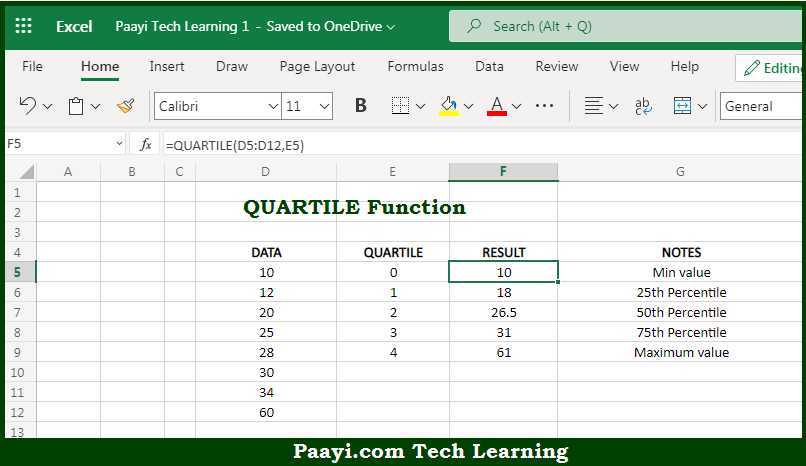# Learn How to Use Microsoft Excel QUARTILE Function

Written by | 0 Comments | 644 Views

In this article, you will learn how to use the Microsoft Excel QUARTILE function and its prime function in Microsoft Excel. You will also get to know the Microsoft Excel QUARTILE function return value and syntax with the help of some examples.

Microsoft Excel QUARTILE Function

The main purpose of the Microsoft Excel QUARTILE function is to get the quartile in the given data set. That implies, with the help of the QUARTILE function you can able to return the quartile of each of four equal groups, for a given set of data. It should be noted that the QUARTILE function can return minimum value, first quartile, second quartile, third quartile, and max value. So, with the help of the QUARTILE function, you can able to get the quartile in the given data set.

Return Value of QUARTILE Function

The return value will be the value for the requested quartile.

Syntax of QUARTILE Function

=QUARTILE(array, quart)

Where the arguments:

• array: This is the reference containing data to analyze.
• quart: This is the quartile value to return.

## How to Use Microsoft Excel QUARTILE Function?So we know that Microsoft Excel QUARTILE function you can able to get the quartile in the given data set. That implies, with the help of the QUARTILE function you can able to return the quartile of each of four equal groups, for a given set of data. It should be noted that the QUARTILE function can return minimum value, first quartile, second quartile, third quartile, and max value. So, with the help of the QUARTILE function, you can able to get the quartile in the given data set.